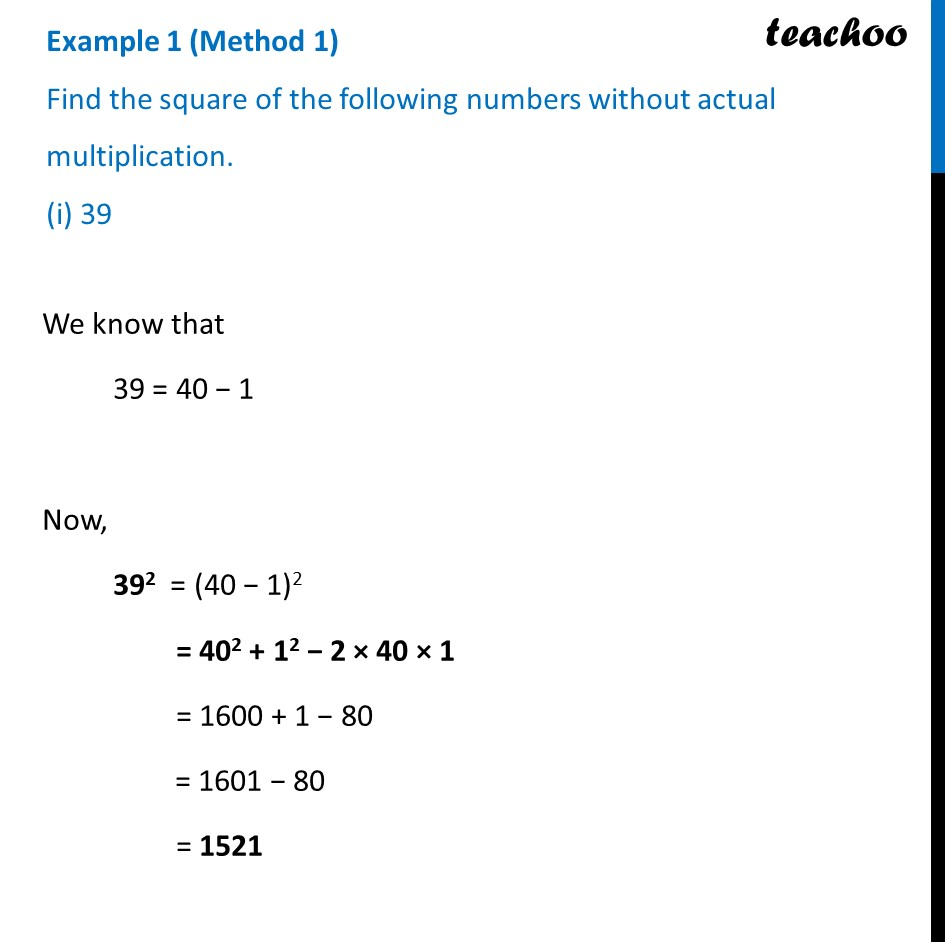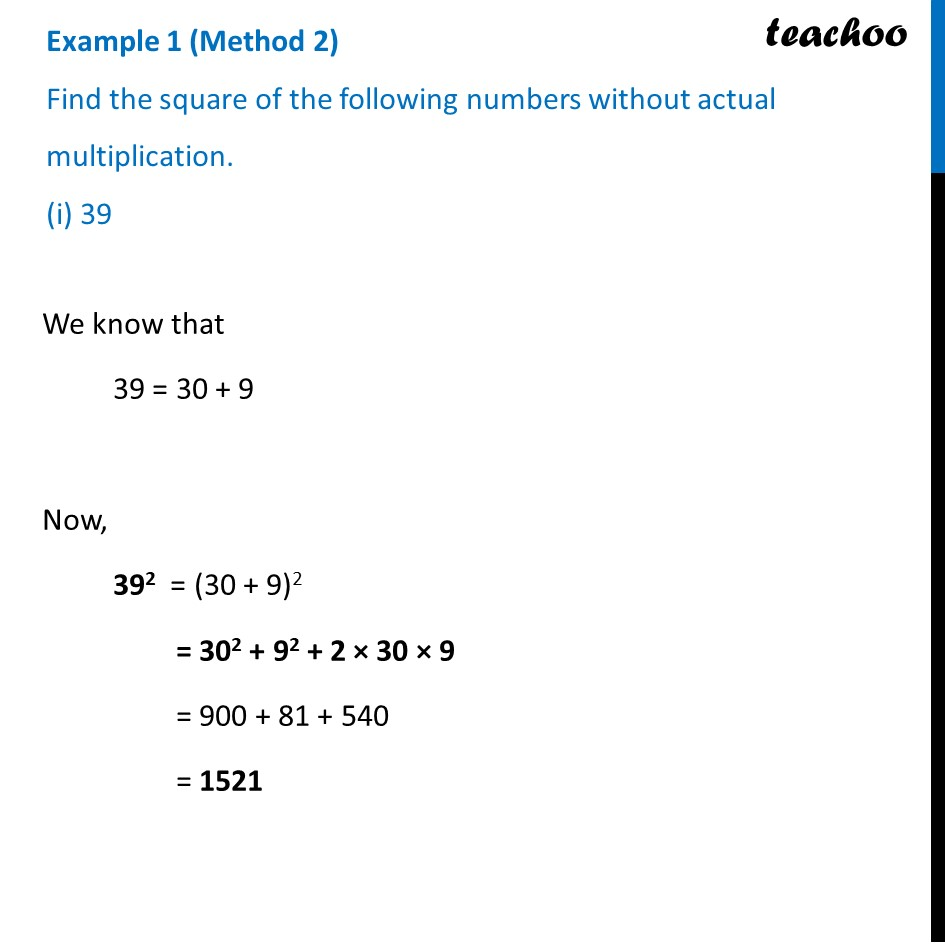Examples

Chapter 5 Class 8 Squares and Square Roots
Serial order wiseLearn in your speed, with individual attention - Teachoo Maths 1-on-1 Class

### Transcript

Example 1 (Method 1) Find the square of the following numbers without actual multiplication. (i) 39 We know that 39 = 40 − 1 Now, 392 = (40 − 1)2 = 402 + 12 − 2 × 40 × 1 = 1600 + 1 − 80 = 1601 − 80 = 1521 Example 1 (Method 2) Find the square of the following numbers without actual multiplication. (i) 39 We know that 39 = 30 + 9 Now, 392 = (30 + 9)2 = 302 + 92 + 2 × 30 × 9 = 900 + 81 + 540 = 1521